# What is a CI?

• Using a Statistic to estimate a Parameter

• It is NOT a probability

• It is an interval that will cover the true parameter X% of the time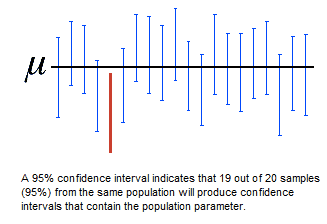• So we can interpret a CI as

• "We are X% confident that the true population parameter lies within A and B"

# General Math Behind a CI

• Formula

• Point Estimate ± Margin of Error

• Point Estimate ± Critical Value * Standard Error• Point Estimate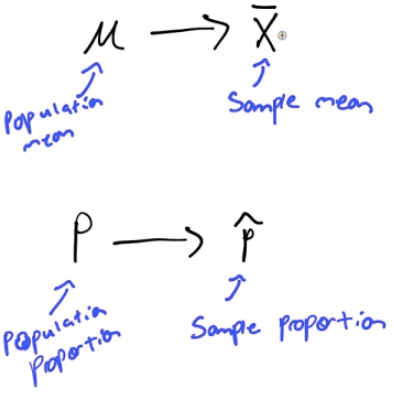• Critical Value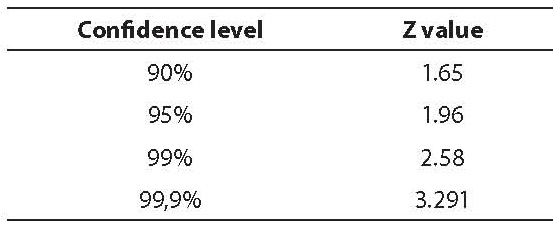• Standard Error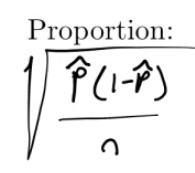• Confidence Interval# Steps to Calculating a CI

• Read the problem and outline the STASTICS

• Random

• Independent: N≥10n

• Normal: n>30

• CALCULATE

• Point Estimate ± Critical Value * Standard Error
• INTERPRET

• Some Can Calculate Intervals

# Practice Questions

1. The effect of drugs and alcohol on the nervous system have been the subject of considerable research. Suppose a neurologist is testing the effect of a drug on response time by injecting 50 rats with a dose, subjecting each to a stimulus, and recording the response time. The average response time for the 50 injected rats was 1.26s. Assuming the mean response time for a rat that has not been injected with the drug is 1.4s with standard deviation of 0.45, construct a 90% confidence interval to determine if the drug has an effect on response time.

• Statistics

• Mean = 1.26

• Population SD = 0.45

• n = 50

• CL = 90%

• Conditions

• Random: Assume rats are a random sample

• Independent: N > 10n

• Normal: n > 50

• Calculate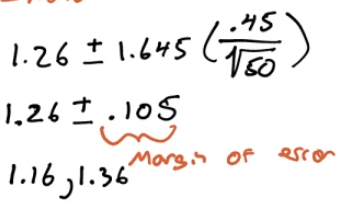• Calculate by calculator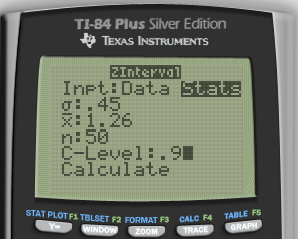• Calculated using Z test
• Interpret

• We are 90% confident that the true mean response time for rats given the new drug is between 1.16s and 1.36s.

• 1.4s is not in the interval, so we have evidence the new drug make rats faster

1. There are two fire stations in a town, one in the northern half and one the southern half. The one in the northern part is known to respond to calls within 4 min. The council members in the town are worried that the southern fire station isn’t as good so they hire a statistician. The statistician collects a random sample of 50 call/responses from the southern fire station. The mean response time is 5.3 min with a standard deviation of 3.1. Construct a 95% confidence interval to determine if the council members have cause to worry about the southern station

• Statistics

• Mean = 3.5

• Sample SD = 3.1

• n = 50

• CL = 95%

• Conditions

• Random: Yes

• Independent: N > 10n = 500

• Normal: n > 30

• Calculate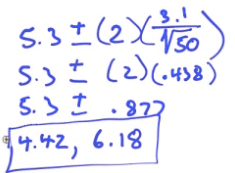• Calculate by calculator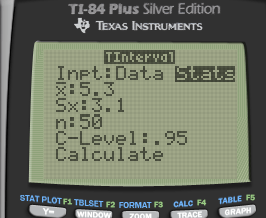![ТИ-84 Pius Stver Editj(n ф TEns [NSTRUMENTS STA1 гоямдтп сам: F4 ](./media/image201.png)

• Interoperate

• We are 95% confident that the true population mean response time for the southern fire station is between 4.42 and 6.18 mins

• 4 is not in the interval, so we do have reason to be concerned.

1. The US Department of Transportation reported that 75% of all fatally injured automobile drivers were intoxicated. A random sample of 32 records in Carson County, Colorado, showed that 16 involved a drunk driver. Use a 99% confidence interval to determine whether or not there is evidence that indicates the population proportion of driver fatalities related to alcohol is different than 75%

• Statistics

• P hat = x / n = 16/32 = 0.5

• n = 32

• CL = 99%

• Conditions

• Random: Yes

• Independent: N > 10n = 320

• Normal: n * p hat > 10 and n * (1-p hat) >10

• Calculate• Calculate by calculator• Interperate

• We are 99% confident that the true population proportion of driver fatalties in Carson County is between 27.2% and 72.8%

• 75% is not in our interval, so it appears that Carson County is lower in the US